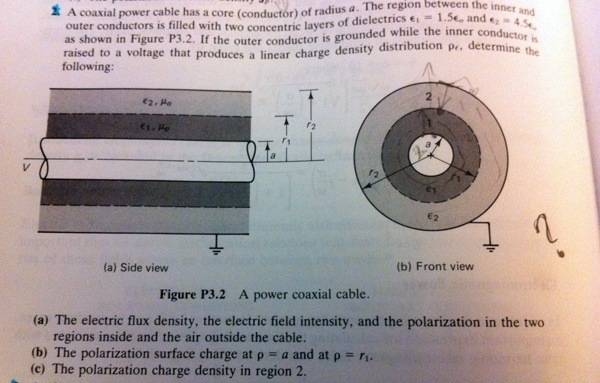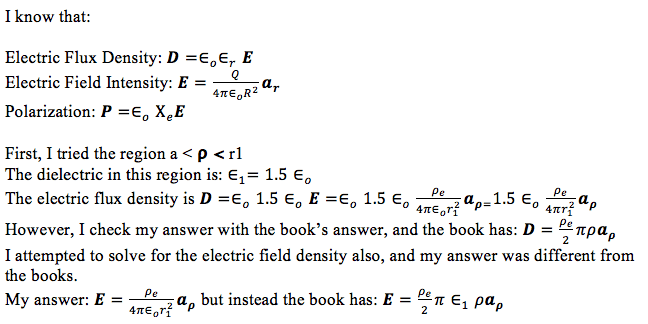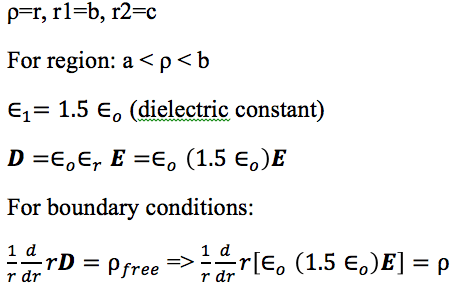# Electric Flux Density for Coaxial Cable

• tquiva
In summary, the problem is that the equation for electric field in cylindrical co-ordinates is not the same as the equation in spherical co-ordinates. The attempted solution suggested using Gauss's law to find the field between points a and c, but this does not seem to be the case. The student is now left without a solution and is requesting help from others.f

## Homework Statement

I am given the following problem:

## The Attempt at a Solution

My known equations and attempt to the problem:

Basically, my answer to the problem is not the same as the book's answer, and I'm pretty sure I'm using the right equations. What could I possibly be doing wrong here? I've been trying to figure this out for three hours now, and am now frustrated. I apologize for my ignorance here, but any advice or tips will be greatly appreciated.

Last edited by a moderator:
The images need a login to access.

Sorry about that. This should work now,

## Homework Statement

I am given the following problem:## Homework Equations

3. The Attempt at a Solution [/B]

My known equations and attempt to the problem:Basically, my answer to the problem is not the same as the book's answer, and I'm pretty sure I'm using the right equations. What could I possibly be doing wrong here? I've been trying to figure this out for three hours now, and am now frustrated. I apologize for my ignorance here, but any advice or tips will be greatly appreciated.

#### Attachments

Since D is radial, have you tried:

$$\frac{1}{r}\frac{d}{dr}r{\bf D} = \rho_{free}$$

... for the appropriate boundary conditions?

(aside: Don't you hate it when people duplicate constant and variable symbols - try $\rho$=r, r1=b and r2=c, ... this allows you to keep $\rho$ values for densities: it'll make discussions easier.)

You will certainly not get an inverse-square relationship as that only happens for spherical symmetry.

Last edited:
I don’t see this equation in my book, but I tried it.I'm not sure where to go from here. Is my equation for E correct?

Could someone please provide me with an example to get me going. My assignment is due very soon and any help is greatly greatly appreciated.

Thank you!

#### Attachments

Google for "electric field cylindrical capacitor" - there's lots.

Using D:
You have to solve the differential equation in general, this will give you some "arbitrary constants" that you find values for from the boundary conditions for each region.

On the inner core surface, you have a charge distribution ... you have a balancing charge distribution on the outer surface, the electric field outside the cable is zero. The D field has to be continuous across all the regeons and you need to know the relationship between D and E.

The equation I gave you was just $\nabla {\bf D} = \rho_{free}$ for cylindrical co-ordinates.

(Note: from gausses law, the field between a and c without the dielectric would be the same as that due to a line of charge right down the middle.)